# The end points of the circuit shown below are connected to a battery and the charge...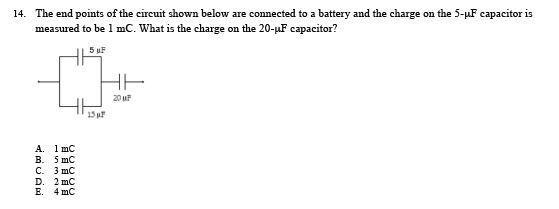The end points of the circuit shown below are connected to a battery and the charge on the 5-mu F capacitor is measured to be 1 mC. What is the charge on the 20-mu F capacitor? A. 1 mC B. 5 mC C. 3 mC D. 2 mC E. 4 mC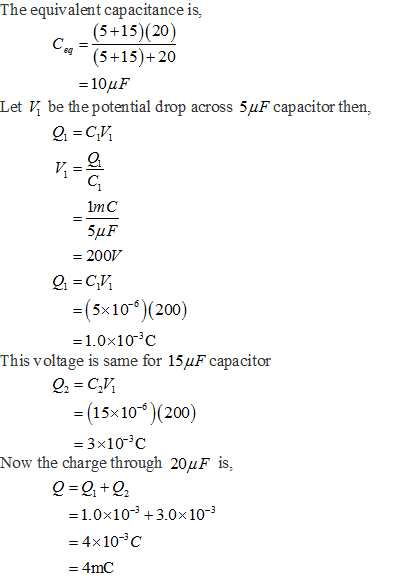#### Earn Coin

Coins can be redeemed for fabulous gifts.

Similar Homework Help Questions
• ### A 12V battery is connected to the capacitor circuit shown What is the total capacitance? What...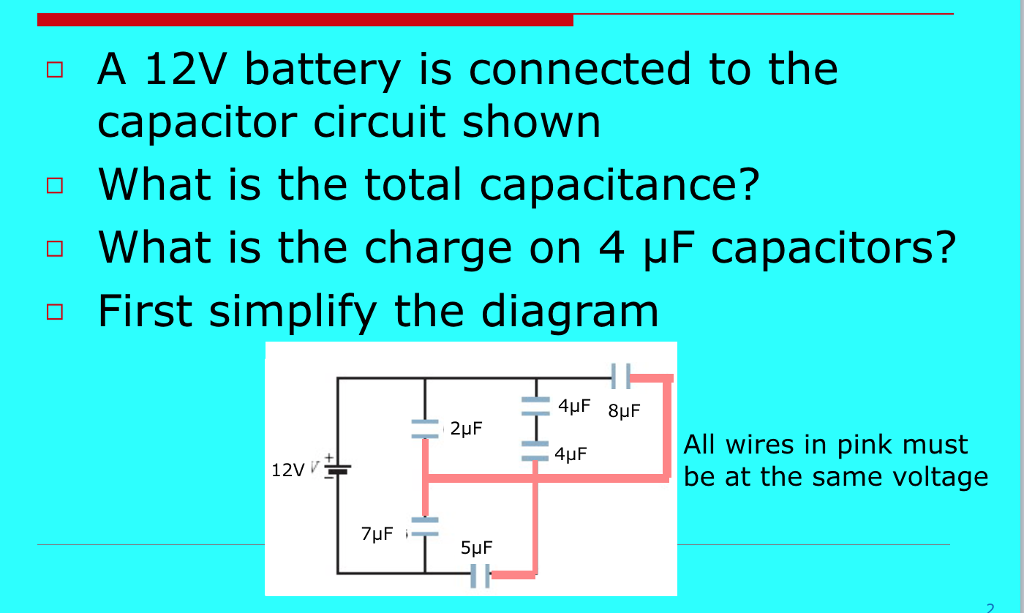A 12V battery is connected to the capacitor circuit shown What is the total capacitance? What is the charge on 4 mu F capacitors? First simplify the diagram

• ### A battery with an emf of 60 {\rm V} is connected to the two capacitors shown...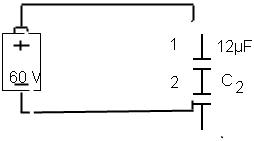A battery with an emf of 60 {\rm V} is connected to the two capacitors shown in the figure . Afterward, the charge on capacitor 2 is 540 \mu C. What is the capacitance of capacitor 2? C2 = ____μF Express your answer using two significant figures. (sorry the immage didnt work well but the 12 uF and C2 are connected in the circuit it just wont line up for me)

• ### In Fig. 25-31, a 20.0 V battery is connected across capacitors of capacitances C_1 = C_6...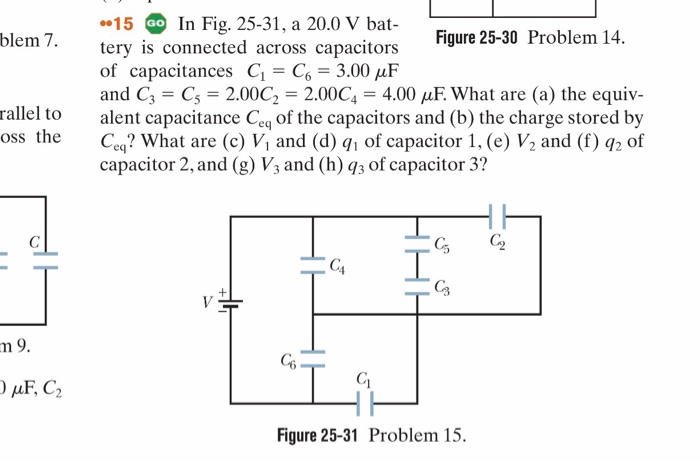In Fig. 25-31, a 20.0 V battery is connected across capacitors of capacitances C_1 = C_6 = 3.00 mu F and C_3 = C_5 = 2.00C_2 = 2.00 C_4 = 4.00 mu F. What are (a) the equivalent capacitance C_eq of the capacitors and (b) the charge stored by C_eq the What are (c) V_1 and (d) q_1 of capacitor 1, (e) V_2 and (f) q_2 of capacitor 2, and (g) V_3 and (h) q_3 of capacitor 3?

• ### The graph below shows the charge on a capacitor as a function of time. The capacitor...

The graph below shows the charge on a capacitor as a function of time. The capacitor is connected in series to a resistor and a battery of emf E = 3 V. Note that the charge is measured in millicoulombs (mC). Use the rules of thumb discussed in class and what you know about the charging process in a capacitor to determine the following quantities (write the values only rounded off to zero decimal places) : 1. The time constant...

• ### A circuit is constructed with five capacitors and a battery as shown. The values for the...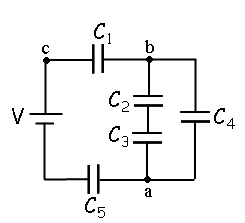A circuit is constructed with five capacitors and a battery as shown. The values for the capacitors are: C1 = C5 = 4.2 μF, C2 = 1 μF, C3 = 5.8 μF, and C4 = 4.4 μF. The battery voltage is V = 12 V. 1) What is Cab, the equivalent capacitance between points a and b?μF 2) What is Cac, the equivalent capacitance between points a and c? 3) What is Q5, the charge on capacitor C5?μC 4) What...

• ### The circuit in the figure below shows four capacitors connected to a battery. The switch S...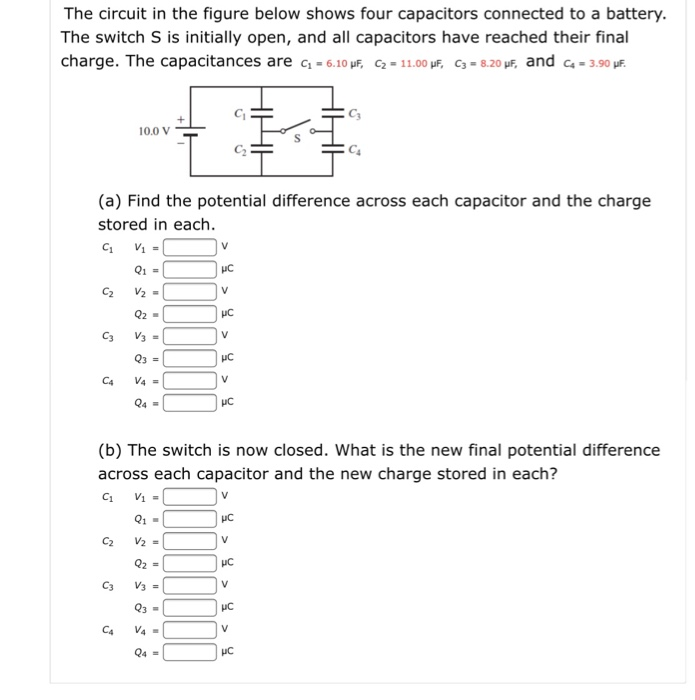The circuit in the figure below shows four capacitors connected to a battery. The switch S is initially open, and all capacitors have reached their final charge. The capacitances are ci-6.10 F, C2. 11.00,F, C3.8.20,f, and ca. 3.90pE 00V (a) Find the potential difference across each capacitor and the charge stored in each HC (b) The switch is now closed. What is the new final potential difference across each capacitor and the new charge stored in each? HC IC Q4-...

• ### Three capacitors are connected to a 12 volt battery as shown above. Given the values of...Three capacitors are connected to a 12 volt battery as shown above. Given the values of the capacitors, find the requested information below. C = 1.72uF C = 4.86F C3 = 2.61uF (A) What is the total capacitance of the circuit in uF? (B) How much energy is stored in the circuit? (C) What is the voltage across each capacitor? 3 III (D) What is the charge on each capacitor in uC? uc ē ū ис a

• ### Consider the circuit below, which shows 3 capacitors charged connected to a 6 volt battery as...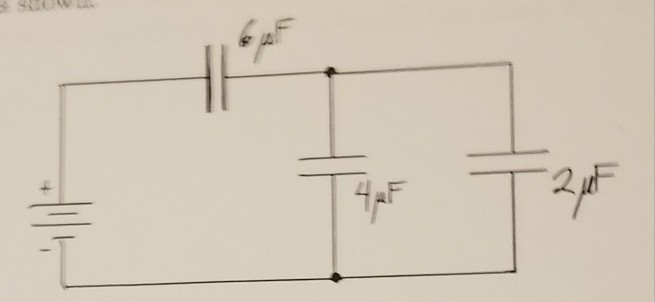Consider the circuit below, which shows 3 capacitors charged connected to a 6 volt battery as shown. a.) what is the potential difference of this network? b.) what is the magnitude of the charge of either plate of the 6 micro farad capacitor Please write neatly and clearly. Thank you.

• ### 4. In the capacitor circuit shown below, what is (A) the equivalent capacitance?i) Start by two...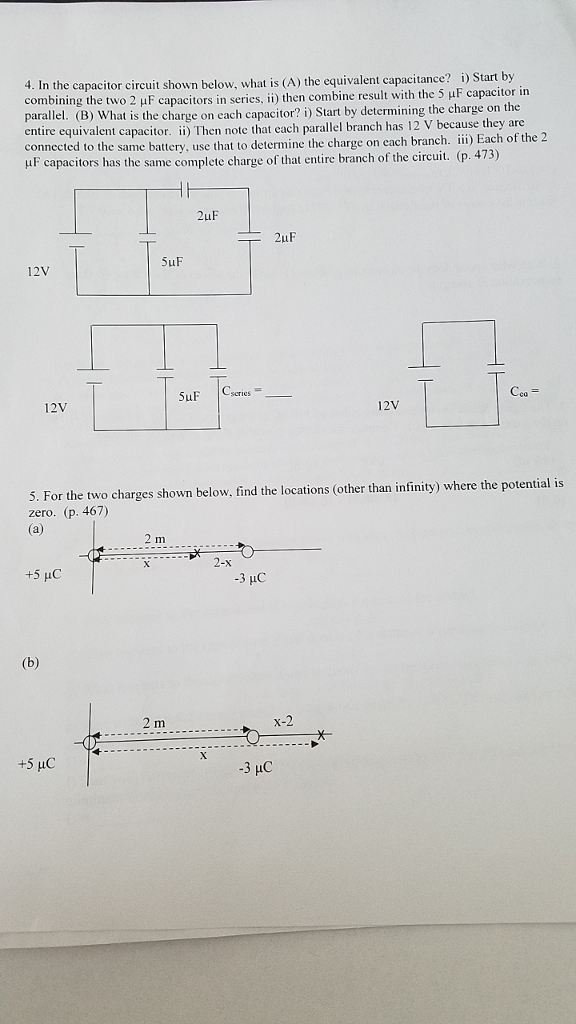4. In the capacitor circuit shown below, what is (A) the equivalent capacitance?i) Start by two 2 μ F capacitors in series, ii) then combine result with the 5 μF capacitor in What is the charge on each capacitor? i) Start by determining the charge on the pacitor. ii) Then note that each parallel branch has 12 V because they are llel. (B) entire equivalent ca connected to the same battery, use that to determine the charge on each branch....

• ### 3. As shown in the circuit below, a capacitor is connected to 2 resistors and battery...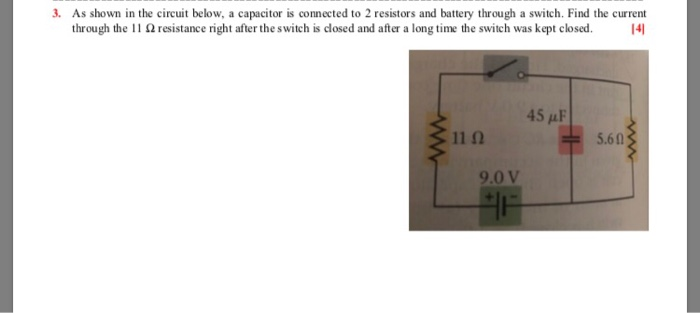3. As shown in the circuit below, a capacitor is connected to 2 resistors and battery through a switch. Find the current through the 11 Ω resistance right after the switch is dosed and after a long time the switch was kept closed. 141 45 AF 11Ω 5.60 9.0 V